### Home > CALC > Chapter 12 > Lesson 12.2.3 > Problem12-92

12-92.
1. Find the derivative with respect to x of each of the following functions and simplify, factoring where possible. Homework Help ✎

1.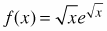2. f(x) = cos kx · sin kx

3.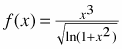4.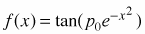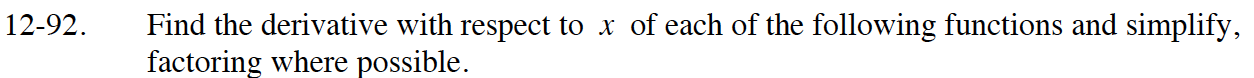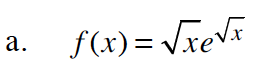$\frac{1}{2}x^{-1/2}e^{\sqrt{x}}+\sqrt{x}e^{\sqrt{x}}\Big(\frac{1}{2}x^{-1/2}\Big)$

$\frac{1}{2}x^{-1/2}e^{\sqrt{x}}+\frac{1}{2}e^{\sqrt{x}}$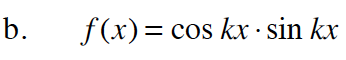$-k\sin(kx)\sin(kx)+k\cos(kx)(\cos(kx)$

$k(\cos^2(x)-\sin^2(x))$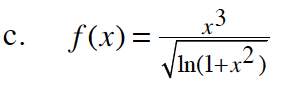$\frac{3x^2\sqrt{\ln(1+x^2)}-x^3\cdot\frac{1}{2}(\ln(1+x^2))^{-1/2}\cdot\frac{2x}{1+x^2}}{\ln(1+x^2)}$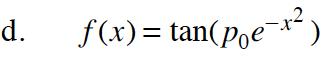$\sec^2(p_0e^{-x^2})(-2xp_0e^{-x^2})$